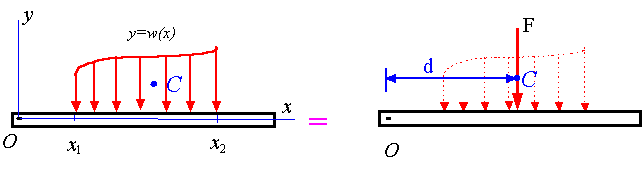Equivalent force systems: Distributed loads

Replacing distributed loads by a resultant load and resultant couple applied at a given point O :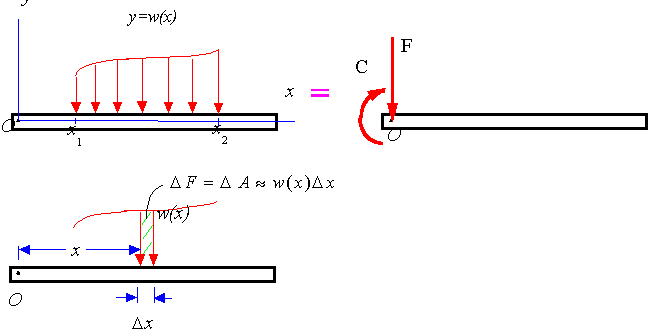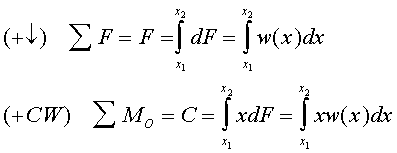Replacing a distributed load by single resultant load: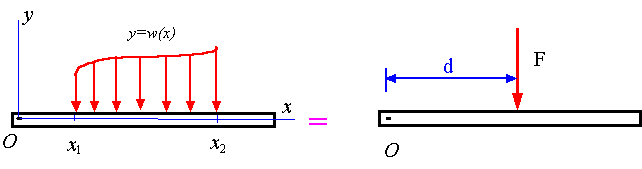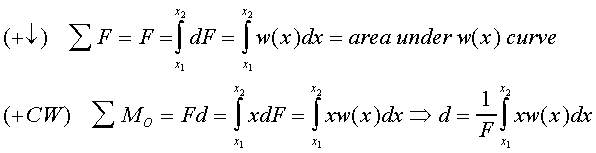Note: Since the equation for d is the same as that for determining the centroid of the area under the w(x) curve, it follows that F must pass through the centroid of the area under the curve w(x).